Ask your WordPress questions! Pay money and get answers fast!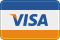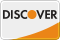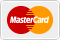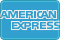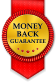# Haversign query sort by distance WordPress

• SOLVED

Hi, I've inherited a WP site built by someone else. It has a property search feature on it, where users can search by location. I have been tasked with allowing them to now sort the results by distance from their search location.

At the moment, if a distance parameter (e.g. within 10 miles) is present in the \$_GET variables, WP is appending this to the query:

" AND ( 3959 * ACOS( COS( RADIANS( ".\$lat." ) ) * COS( RADIANS( latitude.meta_value ) ) * COS( RADIANS( longitute.meta_value ) - RADIANS( ".\$lng." ) ) + SIN( RADIANS( ".\$lat." ) ) * SIN( RADIANS( latitude.meta_value ) ) ) ) < ".floatval( \$_GET["distance"] );

Is there a way to sort this by distance from the search lat/lng? They would like it nearest to furthest away.

2016-02-24

how is this query being constructed? can you show the code please..

There's a lot of it, but the main function is:

```function cgt_find_properties( \$search, \$post_type = "properties", \$rural = false ) { if ( key_exists( "location", \$_GET ) ) { //error_log( \$meta_query ); \$meta_query = cgt_build_meta_query( \$_GET["location"], cgt_query_args() ); } else { \$meta_query = cgt_build_meta_query( \$search, cgt_query_args() ); } \$tax_query = cgt_tax_query( cgt_query_args(), \$post_type, \$rural ); add_filter( 'posts_where' , 'cgt_where_distance' ); //add_filter( 'posts_fields', 'my_geo_fields' ); add_filter( 'posts_join', 'cgt_join_geo_table' ); // Commercial properties don't need this fix because of the way their price is stored and POA being at the top if ( \$post_type != 'commercial_property' ) : add_filter( 'posts_orderby', 'cgt_query_orderby_postmeta_date', 10, 1); else : add_filter( 'posts_join', 'halls_join_freehold' ); add_filter( 'posts_join', 'halls_join_leasehold' ); // Custom ordering with price on application as the highest value add_filter( 'posts_orderby', 'halls_query_orderby_poa', 10, 1); endif; \$paged = (get_query_var('paged')) ? get_query_var('paged') : 1; \$posts_per_page = isset( \$_GET['posts_per_page'] ) ? \$_GET['posts_per_page'] : 12; if ( \$post_type != 'commercial_property' ) : \$ordering = halls_order_by( \$_GET ); else : \$ordering = halls_comm_order_by( \$_GET ); endif; \$args = array( 'post_type' => \$post_type, 'meta_query' => \$meta_query, 'posts_per_page' => \$posts_per_page, 'orderby' => \$ordering["orderby"], 'order' => \$ordering["order"], 'meta_key' => \$ordering["meta_key"], 'paged' => \$paged, 'tax_query' => \$tax_query ); //if ( key_exists( "location", \$_GET ) ) //\$args['areas'] = \$_GET["location"]; if ( key_exists( "property-type", \$_GET ) && !empty( \$_GET["property-type"] ) ) : if ( \$post_type == "properties" && \$rural === false ) { \$args['tax_query'][] = array( 'taxonomy' => 'typeofproperty', "field" => "slug", "terms" => halls_convert_property_type( \$_GET["property-type"] ) ); } else { \$args['typeofproperty'] = \$_GET["property-type"]; } endif; // if rural, there's a reduced set of property types if ( \$rural === true ) { // Stops us overriding choice by the user if ( !key_exists( "property-type", \$_GET ) || empty( \$_GET["property-type"] ) ) : \$args['tax_query'][] = array( 'taxonomy' => 'typeofproperty', "field" => "slug", "terms" => array( "agric-farms-or-land", "land", "property-with-land", "country-home" ) ); else : \$args['tax_query'][] = array( 'taxonomy' => 'typeofproperty', "field" => "slug", "terms" => explode( ",", \$_GET["property-type"] ) ); endif; } //echo \$GLOBALS['wp_query']->request; \$results = new WP_Query( \$args ); if ( \$post_type != 'commercial_property' ) : remove_filter( 'posts_orderby', 'cgt_query_orderby_postmeta_date', 10, 1); else : remove_filter( 'posts_join', 'halls_join_freehold' ); remove_filter( 'posts_join', 'halls_join_leasehold' ); remove_filter( 'posts_orderby', 'halls_query_orderby_poa', 10, 1); endif; remove_filter( 'posts_where' , 'cgt_where_distance' ); //remove_filter( 'posts_fields', 'my_geo_fields' ); remove_filter( 'posts_join', 'cgt_join_geo_table' ); /*echo "<!--"; var_dump( \$results->request ); echo "-->";*/ return \$results; }```

And the filter function is:

```function cgt_where_distance( \$where ) { //Define your context options // \$opts = array( // 'socket' => array( // // IP:PORT use 0 value if you want your operating system to decide // 'bindto' => '5.79.24.197:0', // ) // ); // create the context... \$context = stream_context_create(\$opts); \$fulladdress = iconv( 'UTF-8', 'ASCII//TRANSLIT//IGNORE', halls_sanitise_location( \$_GET["loc"] ).", United Kingdom" ); // new http://theoryapp.com/server-side-geocoding-and-caching/ code \$result = my_geocoding(\$fulladdress); \$lat = \$result['lat']; \$lng = \$result['lng']; /*echo '<!-- google_geocoding = lat:'.\$result["geometry"]["location"]["lat"].', lng:'.\$result["geometry"]["location"]["lng"].' -->'; echo '<!-- my_geocoding = lat:'.\$ret['lat'].', lng:'.\$ret['lng'].' -->';*/ // end /* old \$response = json_decode(file_get_contents( 'https://maps.googleapis.com/maps/api/geocode/json?address=' . rawurlencode( \$fulladdress ) . '&sensor=false&key=AIzaSyAgJ_Xn3gymVZYQgT__8TL9kGrxP5Gqf44', false, \$context, null)); \$coords = \$response->results->geometry->location->lat . ',' . \$response->results->geometry->location->lng; \$lat = \$response->results->geometry->location->lat; \$lng = \$response->results->geometry->location->lng; */ if ( !empty( \$_GET["loc"] ) && !empty( \$_GET["distance"] ) && \$_GET["distance"] > 0 ) { \$where .= " AND ( 3959 * ACOS( COS( RADIANS( ".\$lat." ) ) * COS( RADIANS( halls_latitude.meta_value ) ) * COS( RADIANS( halls_longitute.meta_value ) - RADIANS( ".\$lng." ) ) + SIN( RADIANS( ".\$lat." ) ) * SIN( RADIANS( halls_latitude.meta_value ) ) ) ) < ".floatval( \$_GET["distance"] ); } //echo '<br>'.\$where; return \$where; }```

Taken commented code out of function above:

```function cgt_where_distance( \$where ) { // create the context... \$context = stream_context_create(\$opts); \$fulladdress = iconv( 'UTF-8', 'ASCII//TRANSLIT//IGNORE', halls_sanitise_location( \$_GET["loc"] ).", United Kingdom" ); // new http://theoryapp.com/server-side-geocoding-and-caching/ code \$result = my_geocoding(\$fulladdress); \$lat = \$result['lat']; \$lng = \$result['lng']; if ( !empty( \$_GET["loc"] ) && !empty( \$_GET["distance"] ) && \$_GET["distance"] > 0 ) { \$where .= " AND ( 3959 * ACOS( COS( RADIANS( ".\$lat." ) ) * COS( RADIANS( halls_latitude.meta_value ) ) * COS( RADIANS( halls_longitute.meta_value ) - RADIANS( ".\$lng." ) ) + SIN( RADIANS( ".\$lat." ) ) * SIN( RADIANS( halls_latitude.meta_value ) ) ) ) < ".floatval( \$_GET["distance"] ); } return \$where; }```

this is how to change post order...

``` add_filter( 'posts_orderby', 'edit_posts_orderby', 10, 1 ); function edit_posts_orderby(\$orderby_statement){ \$fulladdress = iconv( 'UTF-8', 'ASCII//TRANSLIT//IGNORE', halls_sanitise_location( \$_GET["loc"] ).", United Kingdom" ); // new http://theoryapp.com/server-side-geocoding-and-caching/ code \$result = my_geocoding(\$fulladdress); \$lat = \$result['lat']; \$lng = \$result['lng']; \$orderby_statement = "( 3959 * ACOS( COS( RADIANS( ".\$lat." ) ) * COS( RADIANS( halls_latitude.meta_value ) ) * COS( RADIANS( halls_longitute.meta_value ) - RADIANS( ".\$lng." ) ) + SIN( RADIANS( ".\$lat." ) ) * SIN( RADIANS( halls_latitude.meta_value ) ) ) ) DESC"; return \$orderby_statement; } ```

you have to choose where to add the filter... you can get some hint on your code.. you can see some "add_filter( 'posts_orderby'" and some "remove_filter( 'posts_orderby'"

you have to check also how their posts_orderby functions are coded... as this may affect the main query and you might not want that to happen... get idea from their functions... but in my code, the line that is most important is this \$orderby_statement = "( 3959 * ACOS( COS( RADIANS( ".\$lat." ) ) * COS( RADIANS(......

Yes, that is part of the issue I was having in that there is an orderby function already. I couldn't see how I could add to this to order by distance. Current function gets the sort_by GET variable.

```function halls_order_by( \$query_string ) { \$mapping = array( "price-low" => array( 'orderby' => "meta_value", 'order' => 'ASC', 'meta_key' => 'price' ), "price-high" => array( 'orderby' => "meta_value", 'order' => 'DESC', 'meta_key' => 'price' ), "recent" => array( 'orderby' => "date", 'order' => 'DESC' ) ); if ( !key_exists( "sort_by", \$query_string ) ) return \$mapping[ 'price-high' ]; return \$mapping[ \$query_string["sort_by"] ]; }```

hmmm... try this one...

maybe we can put your code here...

change this code...
```if ( key_exists( "location", \$_GET ) ) { //error_log( \$meta_query ); \$meta_query = cgt_build_meta_query( \$_GET["location"], cgt_query_args() ); } else { \$meta_query = cgt_build_meta_query( \$search, cgt_query_args() ); }```

to this...
```if ( key_exists( "location", \$_GET ) ) { //error_log( \$meta_query ); \$meta_query = cgt_build_meta_query( \$_GET["location"], cgt_query_args() ); add_filter( 'posts_orderby', 'edit_posts_orderby', 20, 1 ); // change priority to 20.. change to even higher if needed... } else { \$meta_query = cgt_build_meta_query( \$search, cgt_query_args() ); }```

your function edit_posts_orderby much exist somewhere....

Great, it is returning results. It's not easy to see if it is sorting by distance, but it looks promising. What I need to do is only order by distance if the user has chosen that option from the menu. So I guess I need to add a new option to the select menu, then say

``` if(key_exists("location", \$_GET)) { //error_log( \$meta_query ); \$meta_query = cgt_build_meta_query( \$_GET["location"], cgt_query_args() ); if(\$_GET['sort_by'] == 'distance') { add_filter( 'posts_orderby', 'edit_posts_orderby', 20, 1 ); // change priority to 20.. change to even higher if needed... } } ```

yes... that's it..

I also think the right place to add that with the "if(\$_GET['sort_by'] == 'distance')" is above this line \$paged = (get_query_var('paged')) ? get_query_var('paged') : 1;

well, try to arrange the code as needed.. ;)

So the priory of 20 is what overrides the other sort by function below? I think this might have done it. I won't close it just yet in case I need to ask something after testing, if that's okay.

Okay, so I now have:

``` // sort by distance if(\$_GET['sort_by'] == 'distance') { add_filter( 'posts_orderby', 'edit_posts_orderby', 20, 1 ); // change priority to 20.. change to even higher if needed... } \$paged = (get_query_var('paged')) ? get_query_var('paged') : 1; etc... ```

yes.. you're doing it right..

Just doing a few tests, but it's not easy to see if it's working. Is there any way I can echo out the distance value next to each property, so I can see that it's getting gradually further away?

I've also changed the edit_posts_orderby statement so that it sorts ASC rather than DESC, as we want it closest (smallest distance) first. I think that's right?

```function distance_query(\$distance) { \$fulladdress = iconv( 'UTF-8', 'ASCII//TRANSLIT//IGNORE', halls_sanitise_location( \$_GET["loc"] ).", United Kingdom" ); // new http://theoryapp.com/server-side-geocoding-and-caching/ code \$result = my_geocoding(\$fulladdress); \$lat = \$result['lat']; \$lng = \$result['lng']; \$distance .= ", ( 3959 * ACOS( COS( RADIANS( ".\$lat." ) ) * COS( RADIANS( halls_latitude.meta_value ) ) * COS( RADIANS( halls_longitute.meta_value ) - RADIANS( ".\$lng." ) ) + SIN( RADIANS( ".\$lat." ) ) * SIN( RADIANS( halls_latitude.meta_value ) ) ) ) as distance "; return \$distance; } add_filter('posts_fields', 'distance_query');```

and you can var dump the query object to check the result.... you will see additional field, distance... among others like ID, post_author, post_title, etc...

```add_action('template_redirect','test'); function test(){ global \$wp_query; var_dump( \$wp_query->posts ); }```

another thing to note is inside the function cgt_find_properties... at the end you will see a lot of remove filter... you need to add yours too for the post_orderby..

that is

`remove_filter( 'posts_orderby', 'edit_posts_orderby', 20, 1 );`

so that other queries will not use this post orderby..

I would like to suggest also to keep the function and action distance_query.. so that we can change

```function cgt_where_distance( \$where ) { // create the context... \$context = stream_context_create(\$opts); \$fulladdress = iconv( 'UTF-8', 'ASCII//TRANSLIT//IGNORE', halls_sanitise_location( \$_GET["loc"] ).", United Kingdom" ); // new http://theoryapp.com/server-side-geocoding-and-caching/ code \$result = my_geocoding(\$fulladdress); \$lat = \$result['lat']; \$lng = \$result['lng']; if ( !empty( \$_GET["loc"] ) && !empty( \$_GET["distance"] ) && \$_GET["distance"] > 0 ) { \$where .= " AND ( 3959 * ACOS( COS( RADIANS( ".\$lat." ) ) * COS( RADIANS( halls_latitude.meta_value ) ) * COS( RADIANS( halls_longitute.meta_value ) - RADIANS( ".\$lng." ) ) + SIN( RADIANS( ".\$lat." ) ) * SIN( RADIANS( halls_latitude.meta_value ) ) ) ) < ".floatval( \$_GET["distance"] ); } return \$where; }```

to

```function cgt_where_distance( \$where ) { if ( !empty( \$_GET["loc"] ) && !empty( \$_GET["distance"] ) && \$_GET["distance"] > 0 ) { \$where .= " AND distance < " . floatval( \$_GET["distance"] ); } return \$where; }```

and the orderby to

```function edit_posts_orderby(\$orderby_statement){ if ( !empty( \$_GET["loc"] ) && !empty( \$_GET["distance"] ) && \$_GET["distance"] > 0 ) { \$orderby_statement = " distance ASC "; } return \$orderby_statement; }```

and so will just have to do my_geocoding(\$fulladdress); once in a query... unlike before we are doing it twice in 1 query...

Thanks for tidying this up. Although, it now appears to not return any results.

ok... I'm not sure where the fault is... can you check what's working? and maybe we can see what's the problem...

Maybe if I show you the updated function now:

``` function cgt_find_properties( \$search, \$post_type = "properties", \$rural = false ) { if(key_exists("location", \$_GET)) { //error_log( \$meta_query ); \$meta_query = cgt_build_meta_query( \$_GET["location"], cgt_query_args() ); } else { \$meta_query = cgt_build_meta_query(\$search, cgt_query_args()); } \$tax_query = cgt_tax_query( cgt_query_args(), \$post_type, \$rural ); add_filter( 'posts_where' , 'cgt_where_distance' ); add_filter( 'posts_join', 'cgt_join_geo_table' ); // Commercial properties don't need this fix because of the way their price is stored and POA being at the top if ( \$post_type != 'commercial_property' ) : add_filter( 'posts_orderby', 'cgt_query_orderby_postmeta_date', 10, 1); else : add_filter( 'posts_join', 'halls_join_freehold' ); add_filter( 'posts_join', 'halls_join_leasehold' ); // Custom ordering with price on application as the highest value add_filter( 'posts_orderby', 'halls_query_orderby_poa', 10, 1); endif; // sort by distance if(\$_GET['sort_by'] == 'distance') { add_filter( 'posts_orderby', 'edit_posts_orderby', 20, 1 ); // change priority to 20.. change to even higher if needed... add_filter( 'posts_fields', 'add_to_select' ); } \$paged = (get_query_var('paged')) ? get_query_var('paged') : 1; \$posts_per_page = isset( \$_GET['posts_per_page'] ) ? \$_GET['posts_per_page'] : 10; if ( \$post_type != 'commercial_property' ) : \$ordering = halls_order_by( \$_GET ); else : \$ordering = halls_comm_order_by( \$_GET ); endif; \$args = array( 'post_type' => \$post_type, 'meta_query' => \$meta_query, 'posts_per_page' => \$posts_per_page, 'orderby' => \$ordering["orderby"], 'order' => \$ordering["order"], 'meta_key' => \$ordering["meta_key"], 'paged' => \$paged, 'tax_query' => \$tax_query ); //if ( key_exists( "location", \$_GET ) ) //\$args['areas'] = \$_GET["location"]; if ( key_exists( "property-type", \$_GET ) && !empty( \$_GET["property-type"] ) ) : if ( \$post_type == "properties" && \$rural === false ) { \$args['tax_query'][] = array( 'taxonomy' => 'typeofproperty', "field" => "slug", "terms" => halls_convert_property_type( \$_GET["property-type"] ) ); } else { \$args['typeofproperty'] = \$_GET["property-type"]; } endif; // if rural, there's a reduced set of property types if ( \$rural === true ) { // Stops us overriding choice by the user if ( !key_exists( "property-type", \$_GET ) || empty( \$_GET["property-type"] ) ) : \$args['tax_query'][] = array( 'taxonomy' => 'typeofproperty', "field" => "slug", "terms" => array( "agric-farms-or-land", "land", "property-with-land", "country-home" ) ); else : \$args['tax_query'][] = array( 'taxonomy' => 'typeofproperty', "field" => "slug", "terms" => explode( ",", \$_GET["property-type"] ) ); endif; } //echo \$GLOBALS['wp_query']->request; \$results = new WP_Query( \$args ); if ( \$post_type != 'commercial_property' ) : remove_filter( 'posts_orderby', 'cgt_query_orderby_postmeta_date', 10, 1); else : remove_filter( 'posts_join', 'halls_join_freehold' ); remove_filter( 'posts_join', 'halls_join_leasehold' ); remove_filter( 'posts_orderby', 'halls_query_orderby_poa', 10, 1); endif; remove_filter( 'posts_where' , 'cgt_where_distance' ); remove_filter( 'posts_join', 'cgt_join_geo_table' ); // sort by distance if(\$_GET['sort_by'] == 'distance') { remove_filter( 'posts_orderby', 'edit_posts_orderby', 20, 1 ); remove_filter( 'posts_fields', 'add_to_select' ); } /*echo "<!--"; var_dump( \$results->request ); echo "-->";*/ return \$results; } ```

Okay, so now I have:

``` function cgt_find_properties( \$search, \$post_type = "properties", \$rural = false ) { if(key_exists("location", \$_GET)) { //error_log( \$meta_query ); \$meta_query = cgt_build_meta_query( \$_GET["location"], cgt_query_args() ); } else { \$meta_query = cgt_build_meta_query(\$search, cgt_query_args()); } \$tax_query = cgt_tax_query( cgt_query_args(), \$post_type, \$rural ); add_filter( 'posts_where' , 'cgt_where_distance' ); add_filter( 'posts_join', 'cgt_join_geo_table' ); // Commercial properties don't need this fix because of the way their price is stored and POA being at the top if ( \$post_type != 'commercial_property' ) : add_filter( 'posts_orderby', 'cgt_query_orderby_postmeta_date', 10, 1); else : add_filter( 'posts_join', 'halls_join_freehold' ); add_filter( 'posts_join', 'halls_join_leasehold' ); // Custom ordering with price on application as the highest value add_filter( 'posts_orderby', 'halls_query_orderby_poa', 10, 1); endif; // sort by distance if(\$_GET['sort_by'] == 'distance') { add_filter( 'posts_orderby', 'edit_posts_orderby', 20, 1 ); // change priority to 20.. change to even higher if needed... add_filter('posts_fields', 'distance_query'); } \$paged = (get_query_var('paged')) ? get_query_var('paged') : 1; \$posts_per_page = isset( \$_GET['posts_per_page'] ) ? \$_GET['posts_per_page'] : 10; if ( \$post_type != 'commercial_property' ) : \$ordering = halls_order_by( \$_GET ); else : \$ordering = halls_comm_order_by( \$_GET ); endif; \$args = array( 'post_type' => \$post_type, 'meta_query' => \$meta_query, 'posts_per_page' => \$posts_per_page, 'orderby' => \$ordering["orderby"], 'order' => \$ordering["order"], 'meta_key' => \$ordering["meta_key"], 'paged' => \$paged, 'tax_query' => \$tax_query ); //if ( key_exists( "location", \$_GET ) ) //\$args['areas'] = \$_GET["location"]; if ( key_exists( "property-type", \$_GET ) && !empty( \$_GET["property-type"] ) ) : if ( \$post_type == "properties" && \$rural === false ) { \$args['tax_query'][] = array( 'taxonomy' => 'typeofproperty', "field" => "slug", "terms" => halls_convert_property_type( \$_GET["property-type"] ) ); } else { \$args['typeofproperty'] = \$_GET["property-type"]; } endif; // if rural, there's a reduced set of property types if ( \$rural === true ) { // Stops us overriding choice by the user if ( !key_exists( "property-type", \$_GET ) || empty( \$_GET["property-type"] ) ) : \$args['tax_query'][] = array( 'taxonomy' => 'typeofproperty', "field" => "slug", "terms" => array( "agric-farms-or-land", "land", "property-with-land", "country-home" ) ); else : \$args['tax_query'][] = array( 'taxonomy' => 'typeofproperty', "field" => "slug", "terms" => explode( ",", \$_GET["property-type"] ) ); endif; } //echo \$GLOBALS['wp_query']->request; \$results = new WP_Query( \$args ); if ( \$post_type != 'commercial_property' ) : remove_filter( 'posts_orderby', 'cgt_query_orderby_postmeta_date', 10, 1); else : remove_filter( 'posts_join', 'halls_join_freehold' ); remove_filter( 'posts_join', 'halls_join_leasehold' ); remove_filter( 'posts_orderby', 'halls_query_orderby_poa', 10, 1); endif; remove_filter( 'posts_where' , 'cgt_where_distance' ); remove_filter( 'posts_join', 'cgt_join_geo_table' ); // sort by distance if(\$_GET['sort_by'] == 'distance') { remove_filter( 'posts_orderby', 'edit_posts_orderby', 20, 1 ); remove_filter( 'posts_fields', 'distance_query' ); } /*echo "<!--"; var_dump( \$results->request ); echo "-->";*/ return \$results; } ```

so still not returning what we are expecting??

let me see these functions... cgt_where_distance, edit_posts_orderby, distance_query

No results at the mo, although was working before we tidied up the functions. Here is the code:

``` function cgt_where_distance(\$where) { if(!empty(\$_GET["loc"]) && !empty(\$_GET["distance"]) && \$_GET["distance"] > 0) { \$where .= " AND distance < " . floatval( \$_GET["distance"]); } return \$where; } function edit_posts_orderby(\$orderby_statement) { if(!empty(\$_GET["loc"]) && !empty(\$_GET["distance"]) && \$_GET["distance"] > 0) { \$orderby_statement = " distance ASC "; } return \$orderby_statement; } // echo distance value function distance_query(\$distance) { \$fulladdress = iconv( 'UTF-8', 'ASCII//TRANSLIT//IGNORE', halls_sanitise_location( \$_GET["loc"] ).", United Kingdom" ); // new http://theoryapp.com/server-side-geocoding-and-caching/ code \$result = my_geocoding(\$fulladdress); \$lat = \$result['lat']; \$lng = \$result['lng']; \$distance .= ", ( 3959 * ACOS( COS( RADIANS( ".\$lat." ) ) * COS( RADIANS( halls_latitude.meta_value ) ) * COS( RADIANS( halls_longitute.meta_value ) - RADIANS( ".\$lng." ) ) + SIN( RADIANS( ".\$lat." ) ) * SIN( RADIANS( halls_latitude.meta_value ) ) ) ) as distance "; return \$distance; } ```

let's try to remove the "if(!empty(\$_GET["loc"]) && !empty(\$_GET["distance"]) && \$_GET["distance"] > 0)" in the two functions... let's see if that works... let's do something about it later if this works...

function cgt_where_distance(\$where)
{
//if(!empty(\$_GET["loc"]) && !empty(\$_GET["distance"]) && \$_GET["distance"] > 0)
//{
\$where .= " AND distance < " . floatval( \$_GET["distance"]);
//}

return \$where;
}

function edit_posts_orderby(\$orderby_statement)
{
//if(!empty(\$_GET["loc"]) && !empty(\$_GET["distance"]) && \$_GET["distance"] > 0)
//{
\$orderby_statement = " distance ASC ";
//}

return \$orderby_statement;
}

so in these 2 functions, if we put replace back distance with ( 3959 * ACOS( COS( RADIANS( ".\$lat." ) ) * COS( RADIANS( halls_latitude.meta_value ) ) * COS( RADIANS( halls_longitute.meta_value ) - RADIANS( ".\$lng." ) ) + SIN( RADIANS( ".\$lat." ) ) * SIN( RADIANS( halls_latitude.meta_value ) ) ) )

it works? because this is what we have changed..

Does this look right? Sorry, all those brackets are hurting my head.

function cgt_where_distance(\$where)
{
//if(!empty(\$_GET["loc"]) && !empty(\$_GET["distance"]) && \$_GET["distance"] > 0)
//{
\$where .= " AND ( 3959 * ACOS( COS( RADIANS( ".\$lat." ) ) * COS( RADIANS( halls_latitude.meta_value ) ) * COS( RADIANS( halls_longitute.meta_value ) - RADIANS( ".\$lng." ) ) + SIN( RADIANS( ".\$lat." ) ) * SIN( RADIANS( halls_latitude.meta_value ) ) ) ) < " . floatval( \$_GET["distance"]);
//}

return \$where;
}

function edit_posts_orderby(\$orderby_statement)
{
//if(!empty(\$_GET["loc"]) && !empty(\$_GET["distance"]) && \$_GET["distance"] > 0)
//{
\$orderby_statement = " ( 3959 * ACOS( COS( RADIANS( ".\$lat." ) ) * COS( RADIANS( halls_latitude.meta_value ) ) * COS( RADIANS( halls_longitute.meta_value ) - RADIANS( ".\$lng." ) ) + SIN( RADIANS( ".\$lat." ) ) * SIN( RADIANS( halls_latitude.meta_value ) ) ) )";
//}

return \$orderby_statement;
}

haha sorry....

I'm not really good at mySQL.. our SQL was the problem after I've searched google...

we can't use distance in our post where... because it is evaluated first and so distance as a column is unknown...

we need to change only post where...

this one...

``` function cgt_where_distance(\$where){ if(!empty(\$_GET["loc"]) && !empty(\$_GET["distance"]) && \$_GET["distance"] > 0){ \$where .= " AND distance < " . floatval( \$_GET["distance"]); } return \$where; }```

to this

``` function cgt_where_distance(\$where){ if(!empty(\$_GET["loc"]) && !empty(\$_GET["distance"]) && \$_GET["distance"] > 0){ \$where .= " AND ( 3959 * ACOS( COS( RADIANS( ".\$lat." ) ) * COS( RADIANS( halls_latitude.meta_value ) ) * COS( RADIANS( halls_longitute.meta_value ) - RADIANS( ".\$lng." ) ) + SIN( RADIANS( ".\$lat." ) ) * SIN( RADIANS( halls_latitude.meta_value ) ) ) ) < " . floatval( \$_GET["distance"]); } return \$where; }```

Hmm, updated cgt_where_distance but returns 0 results. I've commented out the 'posts_fields', 'distance_query' filter in case it was that, but still the same.

I've sent you a message...

I don't think it's a big issue having that query running twice. Thanks for trying to optimise it anyway.

2016-02-24

You can echo the distance adding a filter to the select

``` add_filter( 'posts_fields', 'add_to_select' ); function add_to_select( \$sel) { \$context = stream_context_create(\$opts); \$fulladdress = iconv( 'UTF-8', 'ASCII//TRANSLIT//IGNORE', halls_sanitise_location( \$_GET["loc"] ).", United Kingdom" ); \$result = my_geocoding(\$fulladdress); \$lat = \$result['lat']; \$lng = \$result['lng']; if ( !empty( \$_GET["loc"] ) && !empty( \$_GET["distance"] ) && \$_GET["distance"] > 0 ) { \$sel.= " , ( 3959 * ACOS( COS( RADIANS( ".\$lat." ) ) * COS( RADIANS( halls_latitude.meta_value ) ) * COS( RADIANS( halls_longitute.meta_value ) - RADIANS( ".\$lng." ) ) + SIN( RADIANS( ".\$lat." ) ) * SIN( RADIANS( halls_latitude.meta_value ) ) ) ) as distance_val" ; } return \$sel; } ```
//In the loop you can echo
\$post->distance_val;

``` function cgt_where_distance(\$where) { \$context = stream_context_create(\$opts); \$fulladdress = iconv( 'UTF-8', 'ASCII//TRANSLIT//IGNORE', halls_sanitise_location( \$_GET["loc"] ).", United Kingdom" ); // new http://theoryapp.com/server-side-geocoding-and-caching/ code \$result = my_geocoding(\$fulladdress); \$lat = \$result['lat']; \$lng = \$result['lng']; if ( !empty( \$_GET["loc"] ) && !empty( \$_GET["distance"] ) && \$_GET["distance"] > 0 ) { \$where .= " AND ( 3959 * ACOS( COS( RADIANS( ".\$lat." ) ) * COS( RADIANS( halls_latitude.meta_value ) ) * COS( RADIANS( halls_longitute.meta_value ) - RADIANS( ".\$lng." ) ) + SIN( RADIANS( ".\$lat." ) ) * SIN( RADIANS( halls_latitude.meta_value ) ) ) ) < ".floatval( \$_GET["distance"] ); } return \$where; } function edit_posts_orderby(\$orderby_statement) { if(!empty(\$_GET["loc"]) && !empty(\$_GET["distance"]) && \$_GET["distance"] > 0) { \$orderby_statement = " distance_val ASC "; } return \$orderby_statement; } ```